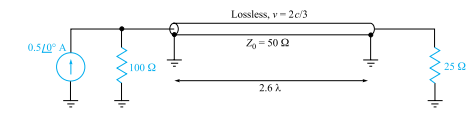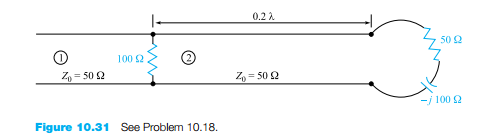### Create an Account

Home / Questions / Determine s on the transmission line of Figure 1032. Note that the dielectric is air b Fin...

# Determine s on the transmission line of Figure 1032. Note that the dielectric is air b Find the input impedance c If ωL 10 Ω find Is d What value of L will produce a maximum value for

Determine s on the transmission line of Figure 10.32. Note that the dielectric is air. (b) Find the input impedance. (c) If ωL = 10 Ω, find Is. (d) What value of L will produce a maximum value for |Is| at ω = 1(b)    Grad/s? For this value of L, calculate the average power (e) supplied by the source; ( f ) delivered to ZL = 40 + j30 Ω.

Jun 14 2020 View more View LessSubscribe To Get Solution